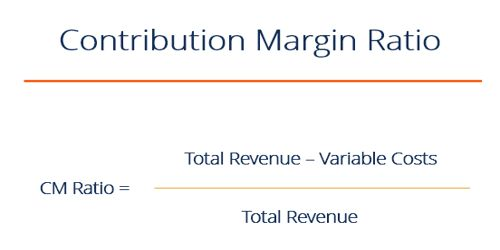# Product’s Contribution Margin Ratio

Product’s Contribution Margin Ratio: Product Contribution margin ratio is commonly expressed as a percentage of sales prices. It is the difference between a company’s sales and variable expenses, expressed as a percentage. It is one of the most important ratios for studying the profitability of operations of a business and establishes the relationship between contribution and sales. A higher ratio means a greater profitability and vice versa.

To calculate the contribution margin ratio, divide the contribution margin by sales. The contribution margin is calculated by subtracting all variable costs from sales. The formula is:

(Sales – Variable expenses) ÷ Sales

Where contribution margin = sales – variable costs.

To calculate the contribution margin that is used in the numerator in the preceding calculation, subtract all variable costs from revenues. So, the contribution margin ratio is the percentage of revenues that are available to cover a company’s fixed costs, fixed expenses, and profit.